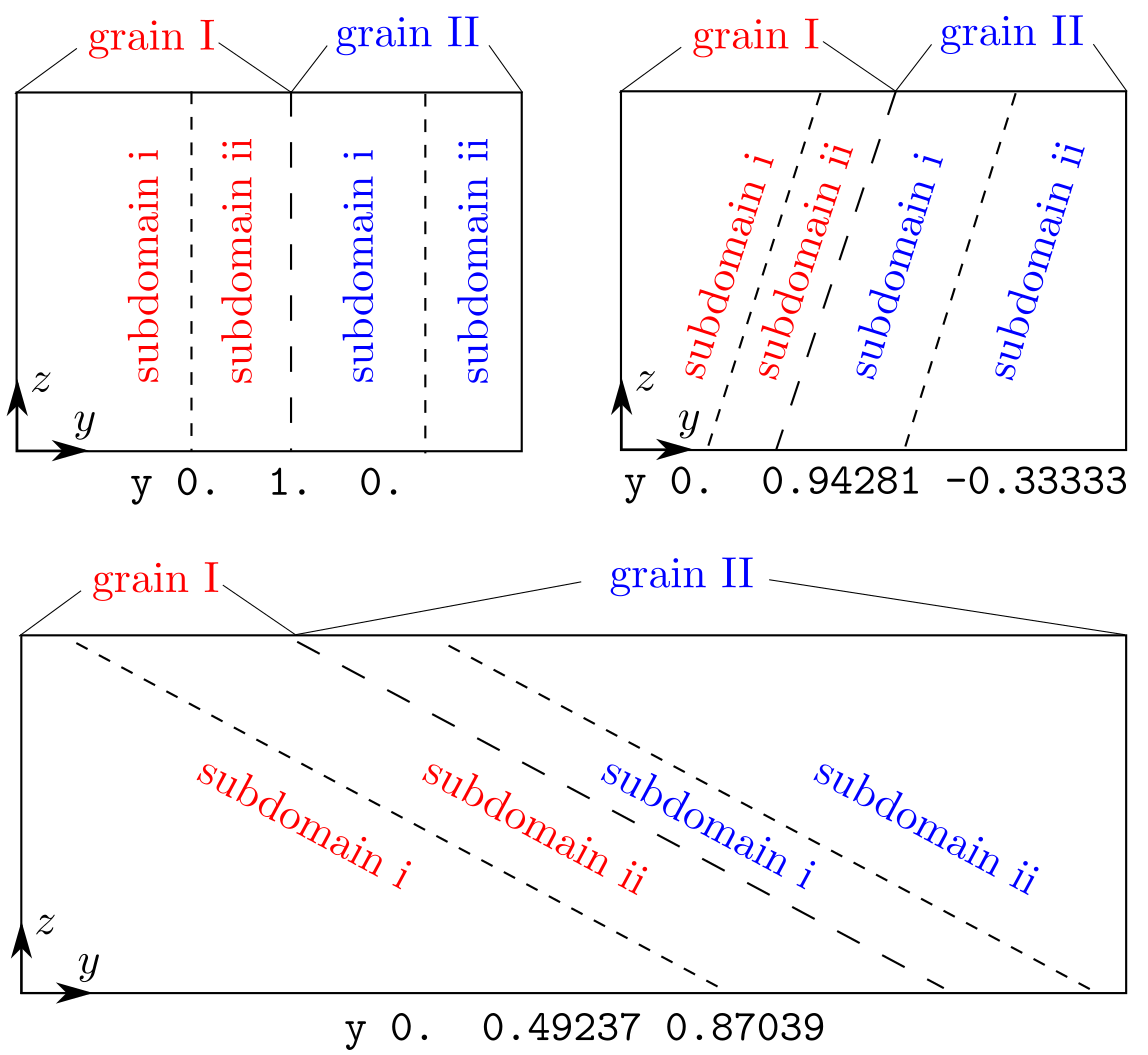# box_dir

### Syntax

box_dir x i j k y i j k z i j k

• i, j, k = real number

### Examples

box_dir x 1. 0. 0. y 0. 1. 0. z 0. 0. 1.
box_dir x 1. 0. 0. y 0. 0.94281 -0.33333 z 0. 0. 1.
box_dir x 1. 0. 0. y 0. 0.49237 0.87039 z 0. 0. 1.


### Description

This command sets the orientation of the subdomain interfaces, including the grain boundary (GB) plane and the atomistic/coarse-grained domain interface, with respect to the simulation cell when there is more than one grain, i.e., grain_num > 1. When grain_num = 1, this command does not take effect.

Assume that direction = 2, i.e., the grains are stacked along the y direction, the first example results in a GB plane normal to the y axis; the second example results in a GB plane inclined with respect to the y axis, as shown in the figure below.The [ijk] vector here is similar to those in the group and modify commands.

In the literature, this command was used to create the $\Sigma 3\{111\}$ coherent twin boundary in Fig. 1 of Xu et al. 2016 and Fig. 1(a) of Xu et al. 2017 and the $\Sigma 11\{113\}$ symmetric tilt grain boundary in Fig. 1(b) of Xu et al. 2017.

As opposed to the grain_mat command whose orientations are for the lattice, the orientations in this command are with respect to the simulation cell. One may use the convert command to convert the crystallographic orientation to the simulation cell-based orientation.

This command becomes irrelevant when boolean_restart = t, in which case there is no need for the subdomain information.

model_init.f90, among many

### Default

box_dir x 1. 0. 0. y 0. 1. 0. z 0. 0. 1.